# 2018-01-29 Intermediate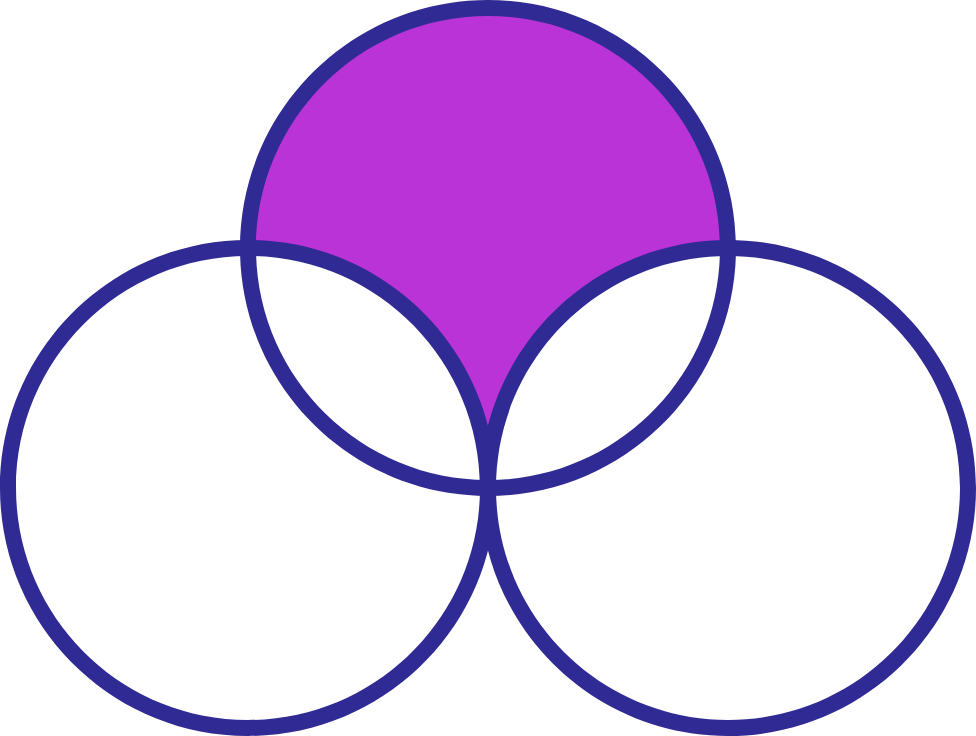I have 3 unit circles. Two of them are externally tangent to each other, and the third one passes through the tangent point, cutting two symmetrical areas from the two circles, as shown above. What is the shaded area?

There are $n$ fish numbered $1$ through $n$ at one end of an aquarium. They all start swimming at the same time to the other end of the aquarium. Once a fish reaches an end of the aquarium, it turns around immediately and continues swimming in the other direction.

Fish $1$ swims with speed $v_1$, fish $2$ swims with speed $v_2 = 2 v_1$, fish $3$ swims with speed $v_3 = 2 v_2$, and so on.

Will the $n$ fish all be at the same end of the aquarium at the same time again?An example aquarium with $n=3$ fish.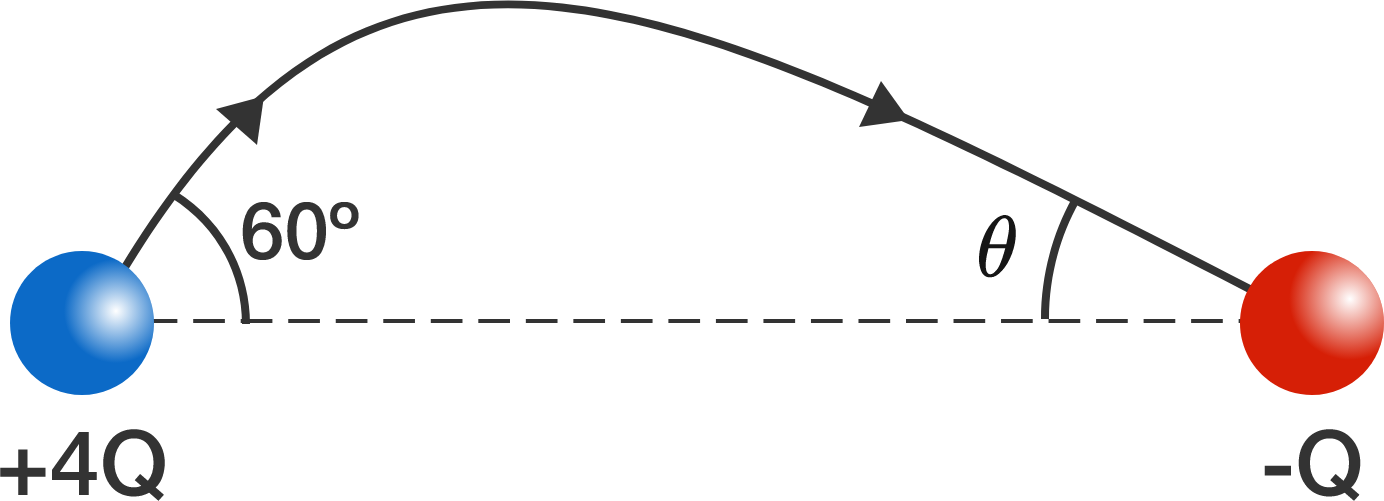Note: the angles in the diagram are not drawn to scale.

An electric field line leaves a positive charge at an angle of $60^{\circ}$ and enters a negative charge at an angle of $\theta$. These angles are measured from the straight line joining the two charges.

The magnitude of the positive charge is 4 times the magnitude of the negative charge.

What is $\theta?$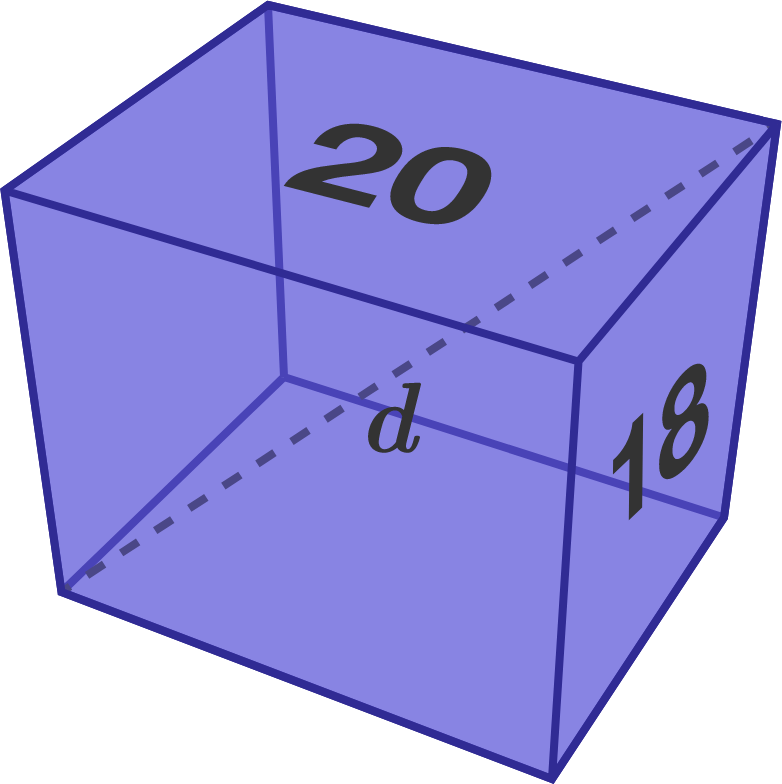Two faces of a rectangular cuboid have areas $20$ and $18.$ Find the minimum possible length of its space diagonal, to three decimal places.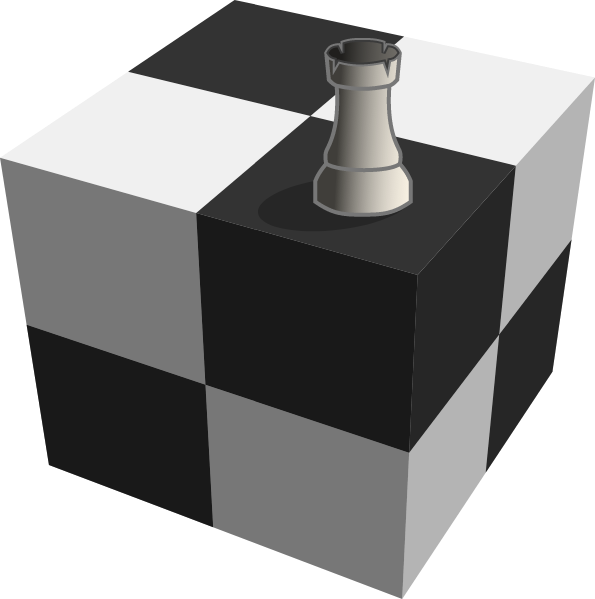Suppose we're playing chess on a $2 \times 2 \times 2$ cube, where the movements of pieces that are considered valid are those that would be valid on a flattened-out net. For example, if a rook is placed on a square, it could reach 13 other squares in one move.

If a queen is placed on a square, how many squares could it reach in one move?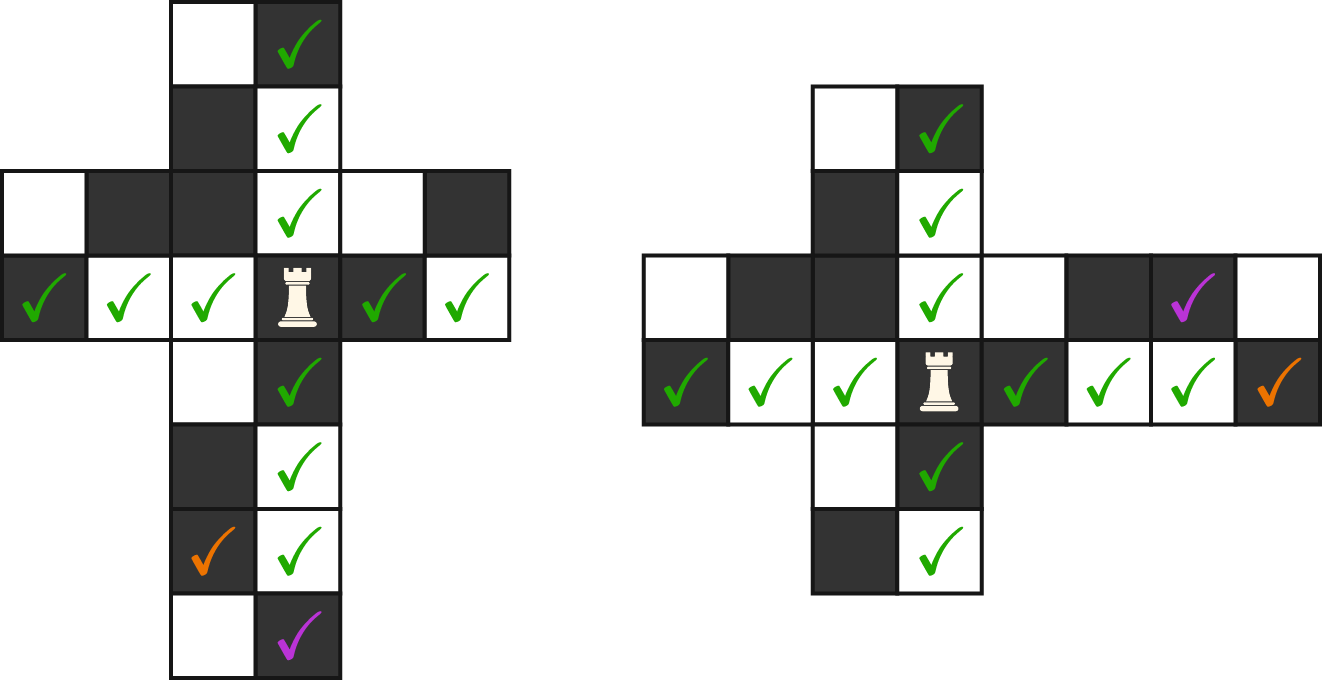The space marked orange is not accessible on the left net, but it is accessible on the right net. Likewise, the space marked purple is accessible on the left net, but is not accessible on the right net. There are 13 accessible spaces in all.

Note: On a $2 \times 2 \times 2$ cube, each of the squares can equivalently be the starting position. As shown above, as long as a space is accessible on at least one net, it is considered a valid movement.

×

Problem Loading...

Note Loading...

Set Loading...